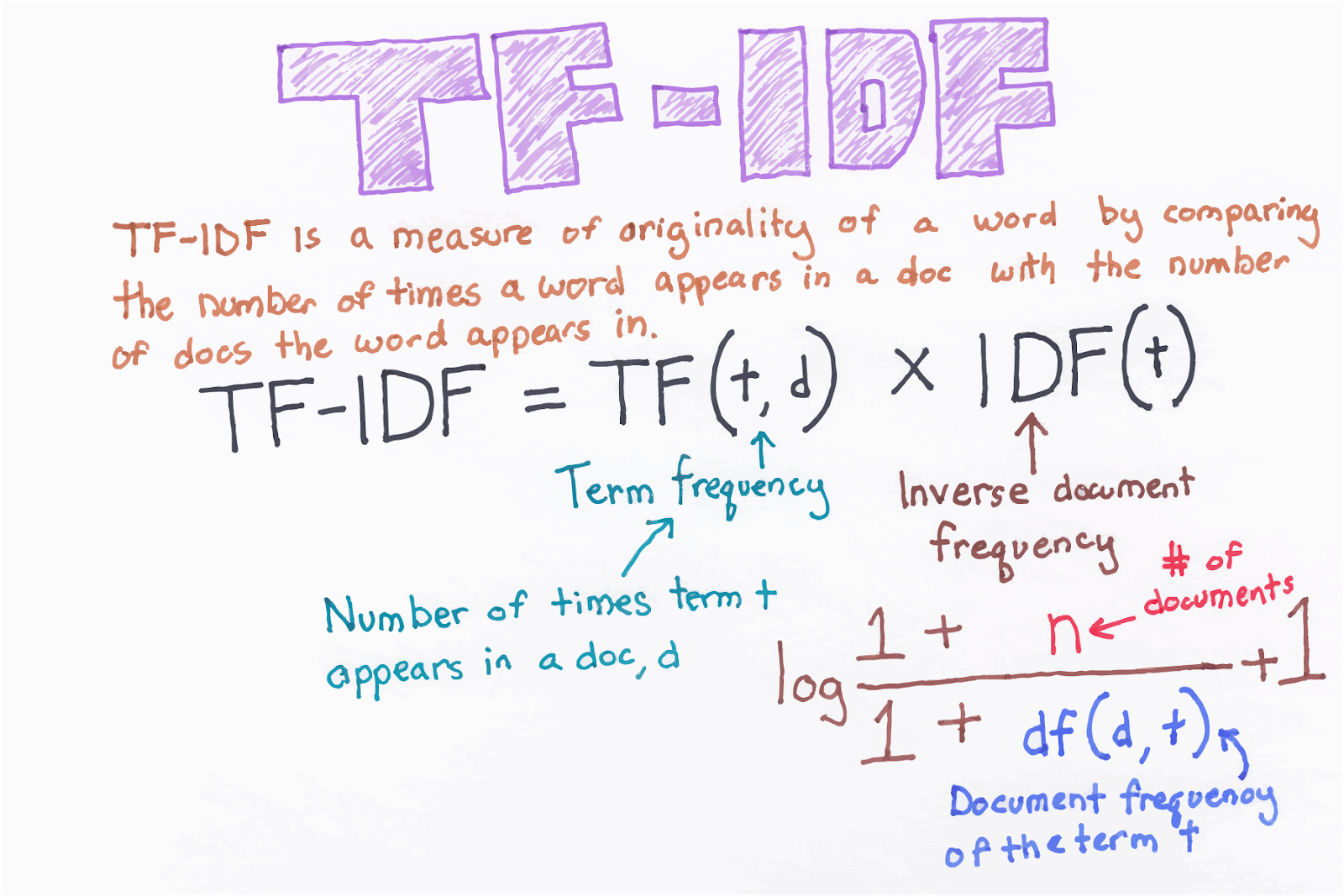# Introduction to Natural Language Processing

Hey - Nick here! This page is a free excerpt from my new eBook Pragmatic Machine Learning, which teaches you real-world machine learning techniques by guiding you through 9 projects.

Natural language processing is a branch of machine learning with the goal of reading, deciphering, and understanding natural human language.

This tutorial will introduce you to basic concepts in natural language processing. We'll move on to building our first natural language processing model in the next tutorial.

## What is Natural Language Processing?

Natural language processing is the field of building computer programs that can read, interact with, and analyze text written in real human languages.

Natural language processing techniques generally have three broad steps:

1. Compile the documents that you'd like to analyze
2. `featurize` the documents - which means calculating quantitative characteristics based on the documents' contents
3. Compare the features of the documents to identify similarities and differences

The first and third steps listed above are relatively simple. It is the `featurize` step where the nuances of natural language processing begin to emerge.

## How To `featurize` Documents in a Natural Language Processing Model

We'll see how to `featurize` documents using Python in the next section of this course. For now, it is still useful to see a very basic example of featurization.

Imagine that you have two very short documents with the following contents:

• `black dog`
• `brown dog`

One common way that you could `featurize` these documents is by creating a Python tuple that contains each unique word in both documents. The tuple could have the generic form of `(brown, black, dog)` with the following values for each document:

• `black dog` - `(0, 1, 1)`
• `brown dog` - `(1, 0, 1)`

A document `featurized` in this manner is often said to be `vectorized`, since this data structure resembles a `vector` from the field of linear algebra.

Similarly, a document represented as a vector of word counts is typically called a `bag of words`. You will see this term again and again in your study of natural language processing models.

Once you have transformed the documents in your data set into bags of words, you can calculate their similarity using cosine similarity. Here is the formula for this metric:## Improving Natural Language Processing Using Term Frequency - Inverse Document Frequency

You can improve on this basic `bag of words` method by adjusting word counts based on their frequency in the body of documents that you are analyzing. This body of documents is sometimes called the `corpus`.

As an example, a high frequency of the words `machine learning` might not be very useful in a `corpus` that contains the lessons frmo this course, since those terms have high frequency in every document.

We use TF-IDF to address this. TD-IDF stands for Term Frequency - Inverse Document Frequency. The `-` is literally the minus sign, while the other two elements of the formula have specific definitions:

• `Term Frequency`: The important of a term within that specific document
• `Inverse Document Frequency` - the importance of that term in the `corpus`

Here are the true mathematical definitions of each term:

• `Term Frequency`: TF(d, t) is the number of occurrences of term `t` in document `d`
• `Inverse Document Frequency` - IDF(t) = log(D/t) where `D` is the total number of documents and `t` is the number of documents within the term

Using these two terms, we can calculate TD-IDF with the following formula:Don't worry if these formulas are a bit difficult to comprehend. It is sufficient to have a high-level understanding of what these terms mean.

## The Use Cases of Natural Language Processing

There are a number of use cases in which natural language processing could prove to be useful. We'll discuss a few of them in this section.

First, imagine that you work for Apple News and you want to curate news. Natural language processing would allow you to recommend similar news articles based on their similarities to an existing article that a user is reading.

Another potential use case of natural language processing is in the legal field. Imagine you're a lawyer working on an obscure legal case and want to find previous rulings that could support your case.

Instead of manually sifting through hundreds of case documents, you could use natural language processing to programmatically find similar cases to the one you're working on.

## Final Thoughts

In this tutorial, you had your first code-free introduction to natural language processing.

Here is a brief summary of what we discussed in this tutorial:

• What natural language processing is
• The steps in building a natural language processing model
• How to `featurize` a document when building a natural language processing model
• Examples of when natural language processing could be useful in the real world